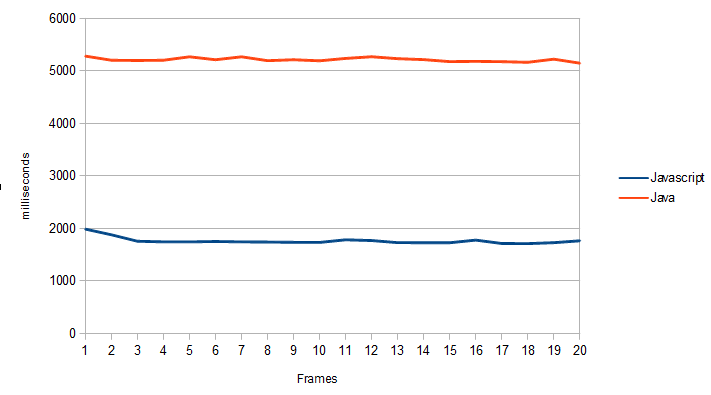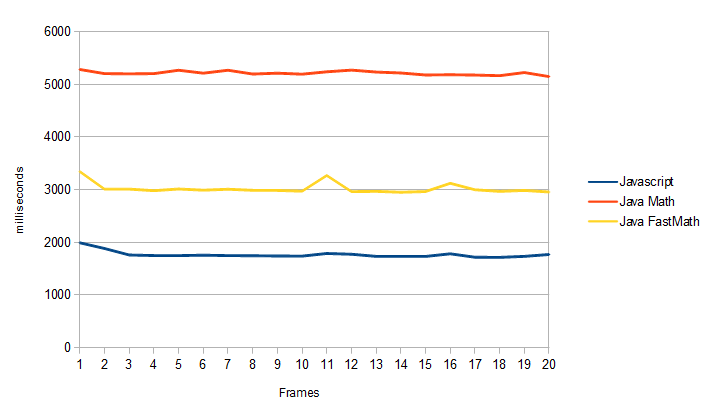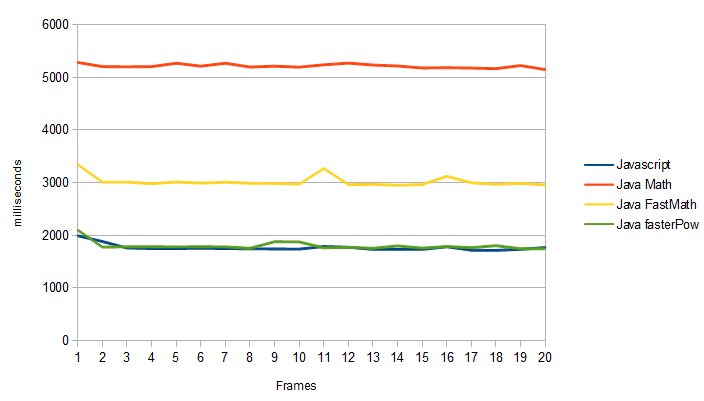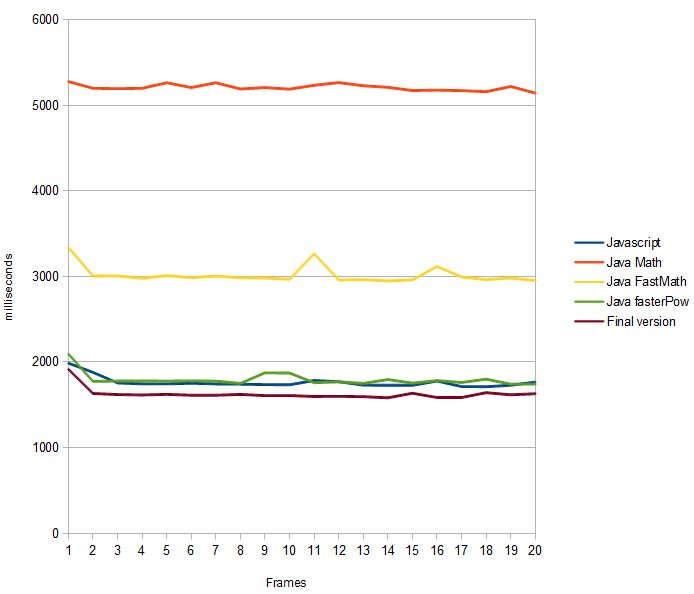# Java vs Javascript: Speed of Math

Last week I’ve created a ray marcher 3d engine which renders the Mandelbulb. And I’ve translated it into pure Javascript a couple of days later. After the translation I decided I should optimize the code a little for speed, so I made some speed improvements in the Javascript code. The main optimization was using an array for the vector3d instead of a class/function.

Rendering the Mandelbulb on a 400x400 canvas now took just 1850ms in Javascript (Chrome, V8). Which is very fast! Even faster than my Java implementation (running on Java 1.6.0.33 -server, which was faster than Java 7). But the Java code didn’t have some of the speed optimizations. So I re-translated the Javascript code back to Java. It produced the following numbers (lower is better performance):What has happened here? The output is the same, why is Java so much slower than Javascript? I would have suspected the opposite…

I fired up the profiler to see what was causing the Java code to be so slow, and it turned out the method it spend most time in was Math.pow(). Other slow methods were Math.acos(), cos(), sin() etc. It turns out that the Math library isn’t very fast, but there is an alternative, FastMath. Apache Commons has implemented a faster Math library for commons-math. Lets see what changing Math.* to FastMath.* does to the performance:This is already much better. But still the method causing most delay is FastMath.pow(). Why is Javascript so much faster? The method is made so you can calculate the power of two doubles, not only integer values. But I’m only doing Integer powers (7 and 8 to be precise). So I decided to implement my own method:

Warning: This isn’t the same as Math.pow/FastMath.pow!The speed with this new method is much better and seems comparable with Javascript. Maybe this is an optimization the V8 engine does by default? Who knows.

The slowest method in the program now is FastMath.acos. From highschool I know that acos(x) can also be calculated as atan(sqrt(1-x*x)/x). So I created a own version of acos. When benchmarked, the different methods: Math.acos(), FastMath.acos() and FastMath.atan(FastMath.sqrt(1-x*x)/x), the result is again surprising:The custom acos() function is a bit faster than FastMath.acos() and a lot faster than Math.acos(). Using this function in the Mandelbulb renderer gives us the following metric:So it turns out that with a bit of tweaking we can get the Java version faster than Javascript, but I would have never imagined Java would be slower in the first place. The Chrome V8 guys really did an amazing job improving the speed of their Javascript VM. Mozilla isn’t far behind, they are getting +/- 2200 ms in the benchmark. Which is also faster than Java.Math and FastMath! It seems that V8’s math implementation has some optimizations that Java could really use. The tricks used above don’t make any difference with the Javascript version.

Edit 1: Is Javascript faster than Java?

Well surprisingly in this case it is. With the code a 100% the same, using arrays as vector and Math.* the code actually runs faster in my browser!

Edit 2: People have been asking me: What could have been done to make it faster in Java? And, why is it slow?